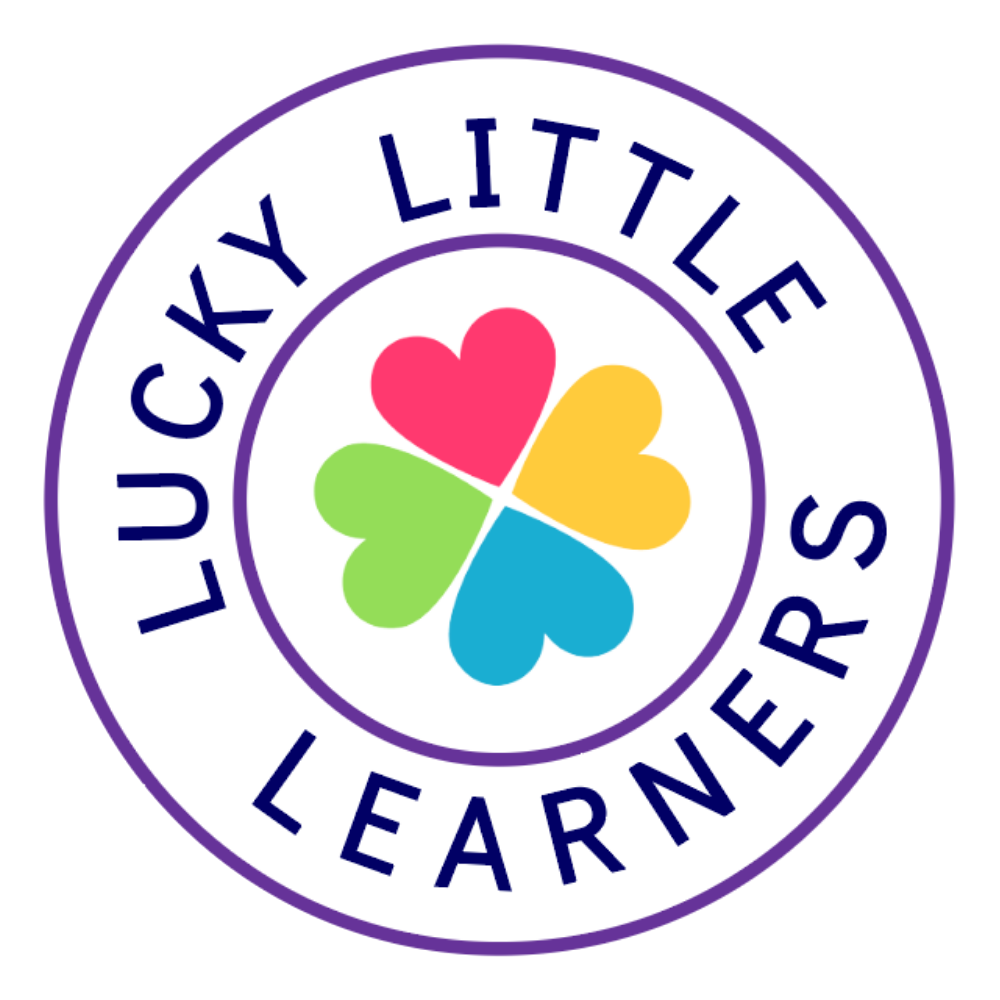Home » Shop » 1st Grade Math Notebook: Subtraction

# 1st Grade Math Notebook: Subtraction

\$6.00

Total Pages: 63
File Size: 5 MB

PREVIEW

This Interactive Math Notebook is designed just for First Grade! The activities can serve as a teaching point, review activity, or math skill intervention. Each activity was created with the first grade student in mind. There are NOT a lot of tiny pieces or cumbersome assembly. Each page is easy to cut and assemble. Each activity comes with a photo of the activity assembled.

***SAVE \$\$\$\$ AND GET THE BUNDLE!!!***

In this subtraction unit, your students will be exposed to skills will include subtraction vocabulary words with definitions, subtraction key words, subtraction within 20 to solve word problems, 8 subtraction strategies (subtracting zero, subtracting the same number, counting back, make a model, missing parts, counting up, think doubles, think addition to 10), ten frames, missing parts, number line subtraction, and fact families.

This unit includes the following activities/resources:
-Interactive Resources
-Key Words for Subtraction
-Subtraction Vocabulary Words & Definitions
-Subtraction Strategies
-Subtraction Fact Wheels
-Draw and Solve
-Counting Back
-Snap Cube Subtraction
-Number Line Subtraction
-Missing Parts
-Part Part Whole
-Draw and Subtract
-Fact Families
-Spin and Subtract
-Ten Frame Subtraction
-Mystery Number
-Story Problem Mats
-Each page includes a photo of the activity assembled
-Dice, Part Part Whole Mat, and Ten Frames are included

This notebook is a wonderful way for students to reference their learning from previous skills, a nice portfolio to show parents at conferences, and a great summary of math skills that have been learned throughout the year.

You can download the preview to see all that is included in this pack. Thanks so much.

CHECK OUT ALL OF MY 1ST GRADE MATH NOTEBOOKS:

Money
-Skills will include coin identification, coin values, adding coins, penny, nickel, dime, quarter, money word problems, coin poems, and how to make different coin combinations

-Skills will include addition vocabulary words with definitions, addition key words, addition within 20 to solve word problems, addition situations (adding to, taking from, putting together), 8 addition strategies (zero facts, turn-around facts, counting on, doubles facts, near doubles, make a ten, part part whole, combine numbers), missing addends, and relate counting to addition

Subtraction
-Skills will include subtraction vocabulary words with definitions, subtraction key words, subtraction within 20 to solve word problems, 8 subtraction strategies (subtracting zero, subtracting the same number, counting back, make a model, missing parts, counting up, think doubles, think addition to 10), ten frames, missing parts, number line subtraction, and fact families

Measurement & Data
-Skills will include types of measurement, tools to measure, ordering objects by length and height, organizing and representing data (tally marks, pictographs, and bar graphs), interpreting data, digital, analog, hour hand, minute hand, labeling minutes on a clock, practice using an interactive clock to tell time, telling time to the hour, half-hour, and quarter-hour

Numbers & Operations
-Skills will include counting to 120, reading and writing numbers to 120, counting forward from any number and ten frames / twenty frames, 1 more 1 less, greater than, less than, and equal to, missing numbers, part part whole, number bonds, and decomposing numbers, base ten, tens and ones, expanded form, groups of ten, and values of digits.

Geometry
-Skills will include equal and unequal parts, partitioning shapes, identifying and writing fractions, 2D and 3D shapes, real world shapes, drawing shapes, determining shape characteristics (sides, corners, edges, vertices, and faces), composing and decomposing shapes, ways shapes move (slides, flips, and turns), congruent shapes, and symmetry.